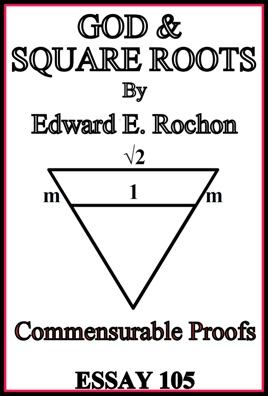• 0,99 €

## Descrizione dell’editore

A brief preface explains the purpose of the essay. Chapter 1 starts by creating a trivial solution to the ratio of √2/1 in the form (Ax + Bx)/1 = √2 with (x) the commensurable, A the coefficient multiplier needed to sum (x) to 1, B the coefficient needed to obtain the product equal to the mantissa of √2 by (x). I then project an equilateral unit triangle (sides = 1) on to the √2 equilateral triangle (sides = √2). I note that line segments (m) equal to the mantissa extend from the unit side to the root side. We note that all individual lines are divisible into commensurable parts through any number of divisions by integer values. We note that any commensurable (x) can be added to the unit line, one on either side, such that (Ax + 2x = s' and (s') is commensurable to (s) (unit length) by definition. Providing we remain within acceptable value limits between √2 and 1 for the commensurable, we can find lines across the triangle anywhere we please that are proportional and similar to 1 and √2 sides. We ask, why should we not be able to slide up to √2, this being so? The answer is that there is no reason, and we prove the commensurable hypothesis. Inversely, starting from √2, a formula for any value of (x) within limits can find a line between √2 and 1 by: Ax - 2x = s'. Chapter 2 restates my Commensurable Theorem and refutes the argument of Hippasus re: relative primes and both sides of the derived equation even numbered, and unbalanced prime factors re: Euclid's argument. They are both erroneous.